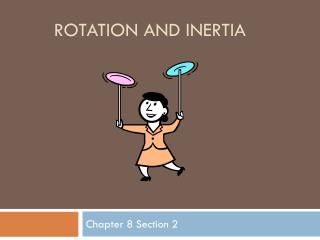DownloadDownload PresentationRotation and Inertia

# Rotation and Inertia

Download Presentation## Rotation and Inertia

- - - - - - - - - - - - - - - - - - - - - - - - - - - E N D - - - - - - - - - - - - - - - - - - - - - - - - - - -
##### Presentation Transcript

1. Rotation and Inertia Chapter 8 Section 2

2. Center of Mass • Center of Mass – The point at which all the mass of the body can be considered to the concentrated when analyzing translational motion. • The special point around which the object rotates neglecting all other forces, except gravity.

3. Rotational and Translational Motion • The complete motion of an object can be described in translational (linear) motion and rotational motion. • Example: • Throwing a hammer into the air. • As the hammer rotates around its center of mass, the hammer travels as a point mass in its parabolic path just as predicted in projectile motion.

4. Parabolic Path of a Projectile • The center of masses of the ball and the rotating bat both follow their parabolic path.

5. Center of Gravity • The center of gravity and the center of mass can be considered the same thing at the basic physics level.

6. Stability • The block topples when the center of gravity extends beyond its support base.

7. Moment of Inertia • Moment of Inertia – The measure of the resistance of an object to changes in rotational motion. • Moment of Inertia depends on both the object’s mass and the distribution of the mass around the axis. • Mass located farther away from the axis has a greater moment of Inertia.

8. Newton’s Second Law • Translational Motion • When a net force acts on an object, the resulting acceleration of the object depends on the objects mass. • Rotational Motion • When a net torque acts on an object, the resulting change in the rotational motion of the object depends on the object’s moment of Inertia.

9. The Moment of Inertia for a Few Shapes

10. The Moment of Inertia for a Few Shapes Cont.

11. Moment of Inertia • Variable for Moment of Inertia = I • Units for Moment of Inertia = kgm2 • Make sure that all units are in SI units when calculating the moment of inertia.

12. Equilibrium • Equilibrium requires both a net force of zero and a net torque of zero • ΣΤ = 0 Nm • ΣF = 0 N • Translational Equilibrium is when the net force is equal to zero. • Rotational Equilibrium is when the net torque is equal to zero.

13. Example Problem #1 • What is the moment of Inertia of a stick around its center of mass if the stick has a mass of 345 grams and a length of 2 feet?

14. Example Problem #1 • I = 1.07x10-2 kgm2

15. Example Problem #2 • An 8.5 m long ladder weighs 350 N. The ladder leans against a frictionless vertical wall. If the ladder makes an angle of 60 degrees with the ground, find the force exerted on the latter by the wall and the force exerted on the latter by the ground.

16. Example Problem #2 Answer • Fx = Rx – Fwall = 0 • Fy = Ry – Weight = 0 • T = -(L/2)(Weight)(Sin30)+(L)(Fwall)(Sin60) = 0 • Fwall = 101.04N • Rx = 101.04N • Ry = 350N • Fground = 364.30N @ 73.90°